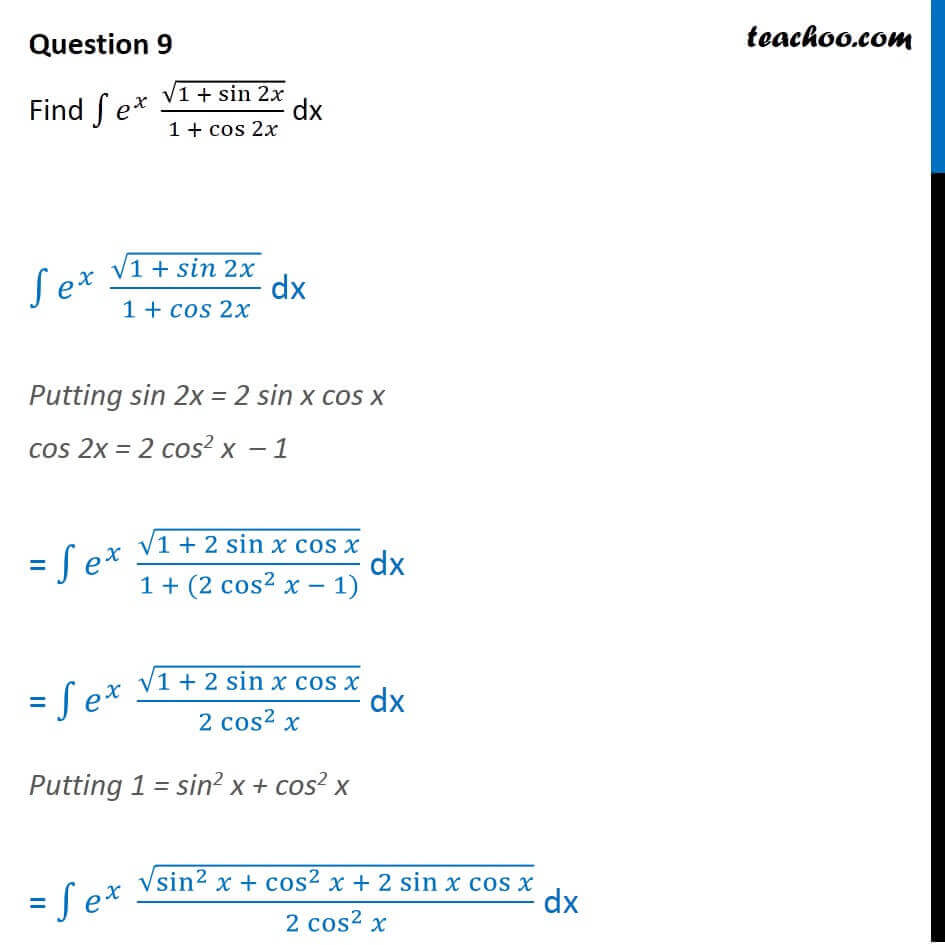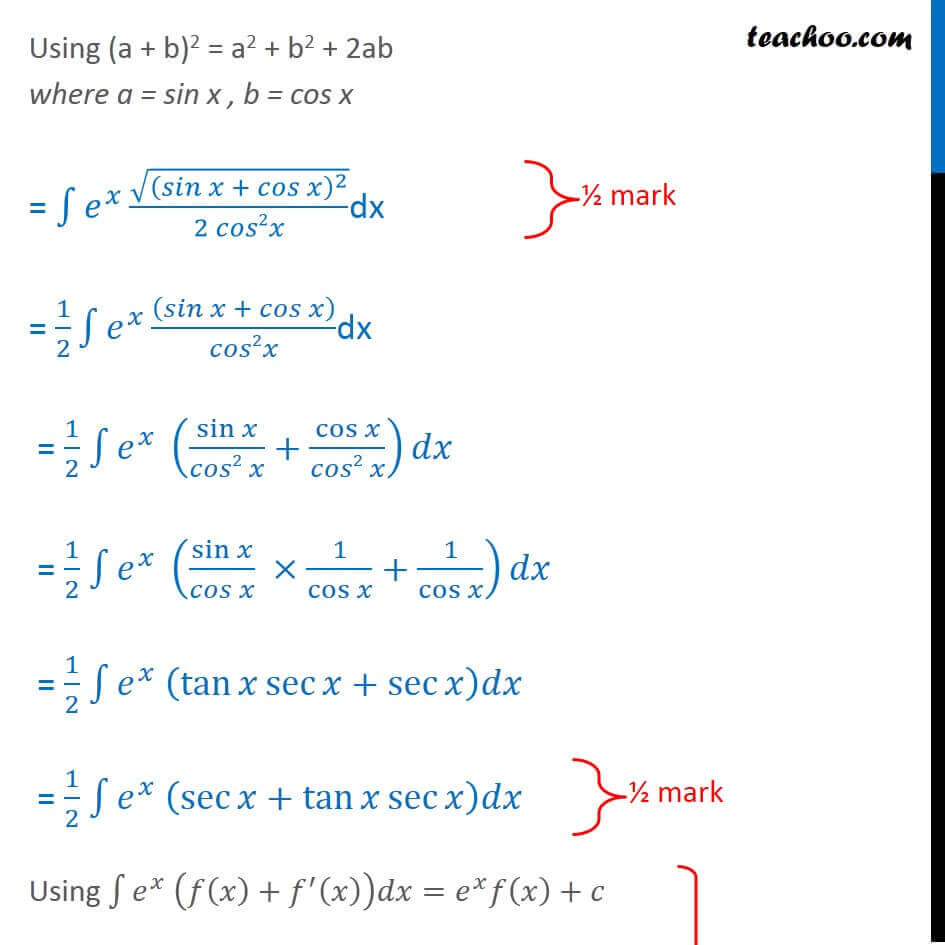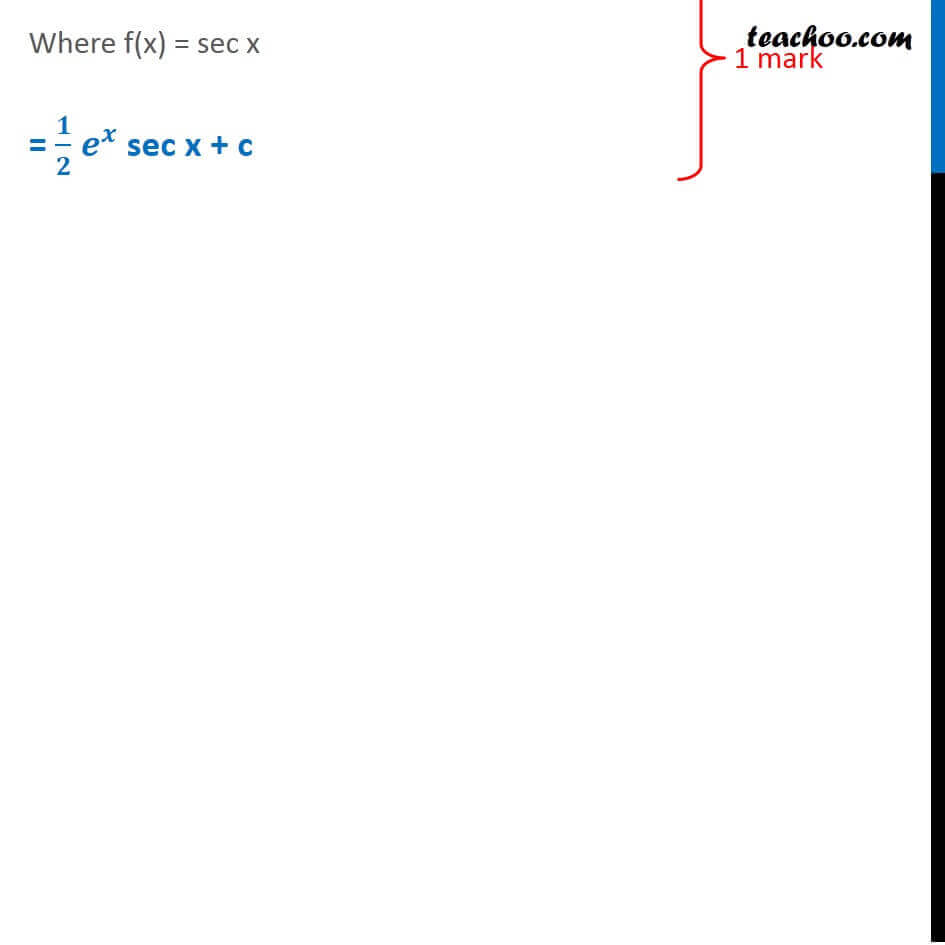### Find ∫e x   √(1 + sin ⁡2x )/(1 + cos ⁡2x ) dx

This is a question of CBSE Sample Paper - Class 12 - 2017/18.1. Class 12
2. Solutions of Sample Papers and Past Year Papers - for Class 12 Boards
3. CBSE Class 12 Sample Paper for 2018 Boards

Transcript

Question 9 Find 1 ^ (1 + sin 2 )/(1 + cos 2 ) dx 1 ^ (1 + 2 )/(1 + 2 ) dx Putting sin 2x = 2 sin x cos x cos 2x = 2 cos2 x 1 = 1 ^ (1 + 2 sin cos )/(1 + (2 cos^2 1)) dx = 1 ^ (1 + 2 sin cos )/(2 cos^2 ) dx Putting 1 = sin2 x + cos2 x = 1 ^ (sin^2 + cos^2 + 2 sin cos )/(2 cos^2 ) dx = 1 (( + )^2 )/(2 2 )dx = 1/2 1 ^ (sin /( 2 )+cos /( 2 )) = 1/2 1 ("sec sec tan x " ) = 1/2 ^ sec x + c [ ^ ( ( )+ ^ ( )) = ^ ( )+ ] Using (a + b)2 = a2 + b2 + 2ab where a = sin x , b = cos x = 1 ^ (( + )^2 )/(2 2 ) dx = 1/2 1 ^ (( + ))/ 2 dx = 1/2 1 ^ (sin /( 2 )+cos /( 2 )) = 1/2 1 ^ (sin /( ) 1/cos +1/cos ) = 1/2 1 ^ (tan sec +sec ) = 1/2 1 ^ (sec +tan sec ) Using 1 ^ ( ( )+ ^ ( )) = ^ ( )+ Where f(x) = sec x = / ^ sec x + c

CBSE Class 12 Sample Paper for 2018 Boards

Class 12
Solutions of Sample Papers and Past Year Papers - for Class 12 Boards